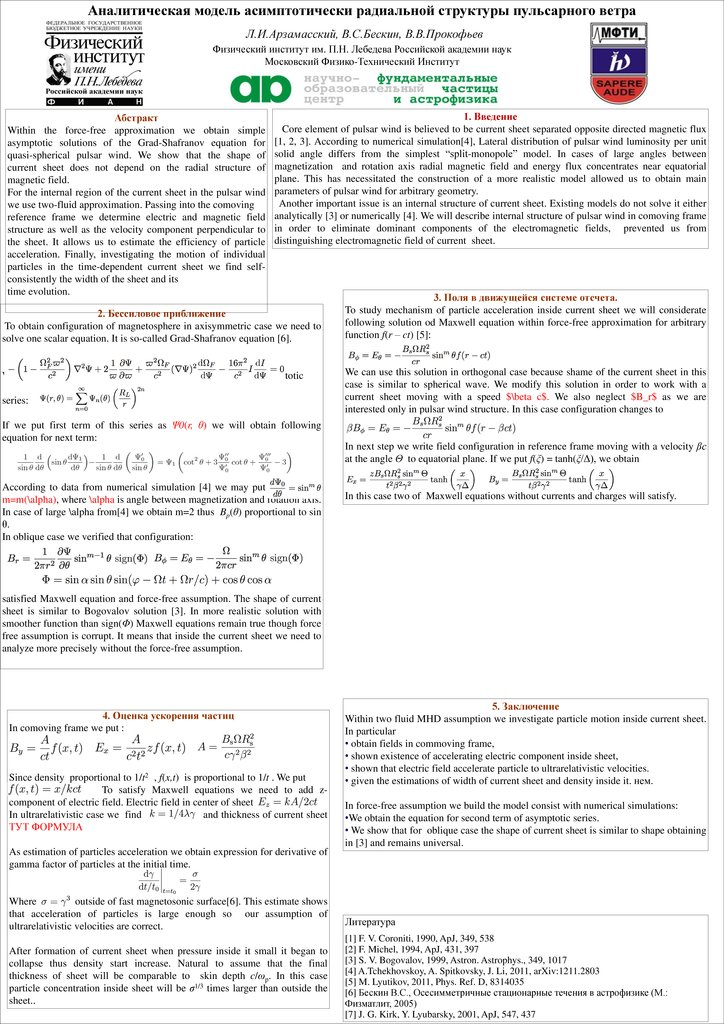# Бессиловое приближение

## 1.

Аналитическая модель асимптотически радиальной структуры пульсарного ветра
Л.И.Арзамасский, В.С.Бескин, В.В.Прокофьев
Физический институт им. П.Н. Лебедева Российской академии наук
Московский Физико-Технический Институт
Абстракт
Within the force-free approximation we obtain simple
asymptotic solutions of the Grad-Shafranov equation for
quasi-spherical pulsar wind. We show that the shape of
current sheet does not depend on the radial structure of
magnetic field.
For the internal region of the current sheet in the pulsar wind
we use two-fluid approximation. Passing into the comoving
reference frame we determine electric and magnetic field
structure as well as the velocity component perpendicular to
the sheet. It allows us to estimate the efficiency of particle
acceleration. Finally, investigating the motion of individual
particles in the time-dependent current sheet we find selfconsistently the width of the sheet and its
time evolution.
1. Введение
Core element of pulsar wind is believed to be current sheet separated opposite directed magnetic flux
[1, 2, 3]. According to numerical simulation, Lateral distribution of pulsar wind luminosity per unit
solid angle differs from the simplest “split-monopole” model. In cases of large angles between
magnetization and rotation axis radial magnetic field and energy flux concentrates near equatorial
plane. This has necessitated the construction of a more realistic model allowed us to obtain main
parameters of pulsar wind for arbitrary geometry.
Another important issue is an internal structure of current sheet. Existing models do not solve it either
analytically  or numerically . We will describe internal structure of pulsar wind in comoving frame
in order to eliminate dominant components of the electromagnetic fields, prevented us from
distinguishing electromagnetic field of current sheet.
2. Бессиловое приближение
To obtain configuration of magnetosphere in axisymmetric case we need to
solve one scalar equation. It is so-called Grad-Shafranov equation .
Where Ψ(r, θ) is magnetic flux. We can search the solution as asymptotic
series:
If we put first term of this series as Ψ0(r, θ) we will obtain following
equation for next term:
According to data from numerical simulation  we may put
,
m=m(\alpha), where \alpha is angle between magnetization and rotation axis.
In case of large \alpha from we obtain m=2 thus Bp(θ) proportional to sin
θ.
In oblique case we verified that configuration:
3. Поля в движущейся системе отсчета.
To study mechanism of particle acceleration inside current sheet we will considerate
following solution od Maxwell equation within force-free approximation for arbitrary
function f(r – ct) :
We can use this solution in orthogonal case because shame of the current sheet in this
case is similar to spherical wave. We modify this solution in order to work with a
current sheet moving with a speed $\beta c$. We also neglect $B_r$ as we are
interested only in pulsar wind structure. In this case configuration changes to
In next step we write field configuration in reference frame moving with a velocity βc
at the angle Θ to equatorial plane. If we put f(ξ) = tanh(ξ/Δ), we obtain
In this case two of Maxwell equations without currents and charges will satisfy.
satisfied Maxwell equation and force-free assumption. The shape of current
sheet is similar to Bogovalov solution . In more realistic solution with
smoother function than sign(Φ) Maxwell equations remain true though force
free assumption is corrupt. It means that inside the current sheet we need to
analyze more precisely without the force-free assumption.
4. Оценка ускорения частиц
In comoving frame we put :
Since density proportional to 1/t2 , f(x,t) is proportional to 1/t . We put
To satisfy Maxwell equations we need to add zcomponent of electric field. Electric field in center of sheet
In ultrarelativistic case we find
and thickness of current sheet
ТУТ ФОРМУЛА
As estimation of particles acceleration we obtain expression for derivative of
gamma factor of particles at the initial time.
Where
outside of fast magnetosonic surface. This estimate shows
that acceleration of particles is large enough so our assumption of
ultrarelativistic velocities are correct.
After formation of current sheet when pressure inside it small it began to
collapse thus density start increase. Natural to assume that the final
thickness of sheet will be comparable to skin depth c/ωp. In this case
particle concentration inside sheet will be σ1/3 times larger than outside the
sheet..
5. Заключение
Within two fluid MHD assumption we investigate particle motion inside current sheet.
In particular
• obtain fields in commoving frame,
• shown existence of accelerating electric component inside sheet,
• shown that electric field accelerate particle to ultrarelativistic velocities.
• given the estimations of width of current sheet and density inside it. нем.
In force-free assumption we build the model consist with numerical simulations:
•We obtain the equation for second term of asymptotic series.
• We show that for oblique case the shape of current sheet is similar to shape obtaining
in  and remains universal.
Литература
 F. V. Coroniti, 1990, ApJ, 349, 538
 F. Michel, 1994, ApJ, 431, 397
 S. V. Bogovalov, 1999, Astron. Astrophys., 349, 1017
 A.Tchekhovskoy, A. Spitkovsky, J. Li, 2011, arXiv:1211.2803
 M. Lyutikov, 2011, Phys. Ref. D, 8314035
 Бескин В.С., Осесимметричные стационарные течения в астрофизике (M.:
Физматлит, 2005)
 J. G. Kirk, Y. Lyubarsky, 2001, ApJ, 547, 437